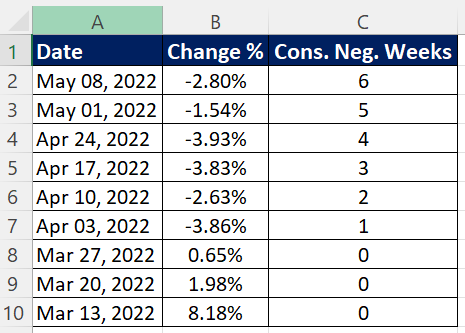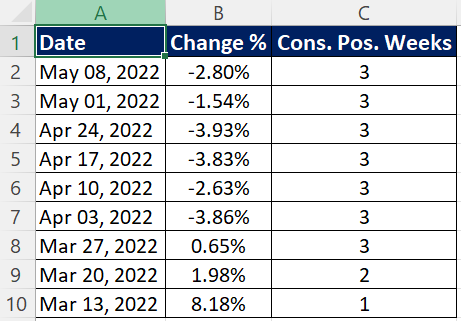# Excel : How to Count Consecutive Positive / Negative Numbers

Suppose you have data consisting of both positive and negative numbers. You need to calculate the number of consecutive negative numbers in Excel. It's easy to count the negative numbers in a list but here we need to focus on the `consecutive` negative numbers. This is a bit challenging problem statement. See the solution below with example.

Example
Let's take a real world example. You have weekly stock market data and you were asked to calculate the number of consecutive weeks market suffered losses.

We have data in column A and B, headers in row 1 and data range starts from cell A2 and ends in cell B10.Enter the formula below in cell C2 and press Ctrl + Shift + Enter to confirm the formula as an array formula.

``=MAX(FREQUENCY(IF(B2:B10<0,ROW(B2:B10)),IF(B2:B10>=0,ROW(B2:B10))))``
Result
There were six consecutive weeks when stock market was in red.How does it work?

Let's understand step by step how it returns the desired result.

Step 1 : Row Numbers of values less than 0
```IF(B2:B10<0,ROW(B2:B10))

returns

{2;3;4;5;6;7;FALSE;FALSE;FALSE}

```
Step 2 : Row Numbers of values greater than or equal to 0
```IF(B2:B10>=0,ROW(B2:B10))

returns

{FALSE;FALSE;FALSE;FALSE;FALSE;FALSE;8;9;10}

```
Step 3 : Array of Consecutive Negative Numbers

Here FREQUENCY function is used to calculate the no. of times negative numbers occur in a row (successively).

```=FREQUENCY({2;3;4;5;6;7;FALSE;FALSE;FALSE},{FALSE;FALSE;FALSE;FALSE;FALSE;FALSE;8;9;10})

returns

{6;0;0;0}

```
Step 4 : Max of the above array

Result is 6 as it was the largest value in the array.

VBA Solution : UDF to count Negative Numbers
The solution below is an alternative to the excel formula shown above. It's a user defined function to compute the consecutive negative numbers. Paste the program below in VBA Editor (press Alt + F11).
```Function ConsNegative(rng As Range)

Dim r As Range
Dim c As Long
Dim m As Long

Application.Volatile
c = 0
m = 0
On Error Resume Next
For Each r In rng.Cells
If r.Value < 0 Then
c = c + 1
If c > m Then
m = c
End If
Else
c = 0
End If
Next r

ConsNegative = m
End Function
```
To make use of the above program, simply enter `=ConsNegative(B2:B10)` in cell C2. It returns the same output as formula.
Excel : Consecutive Positive Numbers
Similarly we can also calculate number of times positive numbers appear consecutively. Formula is almost same as above with the only different in signs. Here we are focusing on positive values.
``=MAX(FREQUENCY(IF(B2:B10>0,ROW(B2:B10)),IF(B2:B10<=0,ROW(B2:B10))))``How to sum the Consecutive Negative Values
The example below is for demonstration purpose only. Please note that adding percentage values doesn't make sense.
```=MIN((COUNTIF(OFFSET(B2:B10,ROW(B2:B10)-ROW(B2),0,C2),"<0")=C2)*SUBTOTAL(9,OFFSET(B2:B10,ROW(B2:B10)-ROW(B2),0,C2)))
```

Enter the formula above in cell D2 and press Ctrl + Shift + Enter to confirm it as an array formula.

Related Posts
Share